Definitions of Square Dance Calls and Concepts
Swing-O-Late [C3B]

From Parallel Waves, Eight Chain Thru, or a Thar. EN: 10
\$BJ?9T\$J(B Wave, Eight Chain Thru, \$B\$^\$?\$O(B Thar \$B\$+\$i(B. JP: 10

Arm Turn 1/2; Centers Trade and Spread as Ends U-Turn Back and Crossover Circulate. EN: 20
Arm Turn 1/2 \$B\$r\$7\$F(B; Centers \$B\$O(B Trade and Spread \$B\$r\$7(B, Ends \$B\$O(B U-Turn Back \$B\$r\$7\$F(B Crossover Circulate \$B\$r\$7\$^\$9(B. JP: 20

Parallel Waves or Eight Chain Thru ends in Parallel Two-Faced Lines. EN: 30
\$BJ?9T\$J(B Wave \$B\$^\$?\$O(B Eight Chain Thru \$B\$+\$i\$O(B, \$BJ?9T\$J(B Two-Faced Line \$B\$G=*\$o\$j\$^\$9(B. JP: 30

Parallel Waves \$B\$+\$i(B: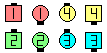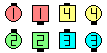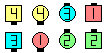Swing-O-Late\$B\$NA0(B Arm Turn 1/2\$B\$N8e(B Centers Trade & Spread as Ends U-Turn Back &Crossover Circulate\$B\$N8e(B (\$B=*\$o\$j(B)

Thar \$B\$+\$i(B: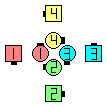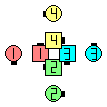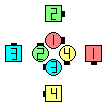Swing-O-Late\$B\$NA0(B Arm Turn 1/2\$B\$N8e(B Centers Trade & Spread as Ends U-Turn Back &Crossover Circulate\$B\$N8e(B (\$B=*\$o\$j(B)

Note: Swing-O-Late is not a R-H call (such as Swing Thru or 1/4 Thru). It can start with either hand. EN: 40
\$BCmSwing-O-Late \$B\$O(B \$B1&JP: 40

anything O-Late [obsolete]:
Do the anything call; Centers Spread as Ends U-Turn Back and Crossover Circulate. EN: 751
anything \$B\$N%3!<%k\$r\$7\$F(B; Centers \$B\$O(B Spread \$B\$r\$7(B, Ends \$B\$O(B U-Turn Back \$B\$r\$7\$F(B Crossover Circulate \$B\$r\$7\$^\$9(B. JP: 751